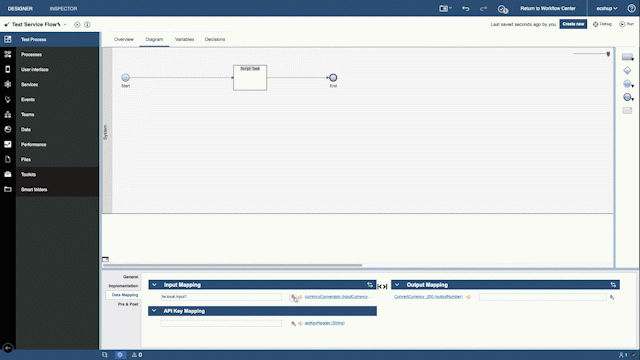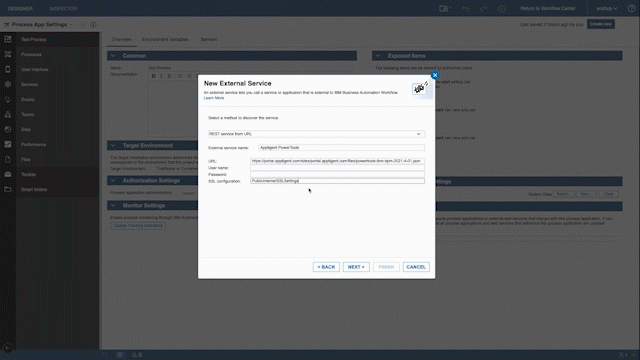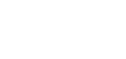## Do more.work Less.

Create advanced business solutions in a fraction of the time with the ultimate automation toolkit for IBM Business Automation Workflow.

## Simple

PowerTools eliminates the need for complex expression syntax and formulas, putting the power to build advanced solutions back in the hands of business users.

No code, no headaches. Just drag, drop, configure and deploy.## Powerful

Redact a sensitive document, localize currencies and dates, translate text into multiple languages, perform advanced calculations, convert text to speech, validate email addresses, shorten hyperlinks, transform data and more with only a few clicks.

## Affordable

PowerTools grows with your business, automatically scaling from just a few users to hundreds of thousands.

Easily add it to your BPM instance in a few simple steps and pay only for what you use with no long-term commitments or contracts.

Pricing plans begin at only \$49/mo.Easy drag-and-drop service task activity support with configurable implementation settings and data mapping configuration.

### Custom Objects

Do your screens and flows rely upon user-driven inputs? That's easy! PowerTools includes a complete set of custom input/output system objects.

### Free Trial

Try PowerTools risk-free for 30 days. Import PowerTools as an external service, then follow the instructions to sign up, subscribe and get an API key.

# Features

Browse the complete list of PowerTools features in the table below. Where available, click on the play icon view a step-by-step tutorial (more tutorials coming soon).

Type
Features
Availability
Add one or more items to an arrayCollectionsRemove from Collection
Remove one or more items from an arrayCollectionsFilter Collection
Search array of strings based on keywordsCollectionsCount Collection
Count the number of items in an arrayCollectionsSort Collection
Sort array of items in ascending or descending orderCollectionsSplit Collection
Split a single array into multiple arraysCollectionsReplace Items
Replace matching items in array with new valueCollectionsContains String
Any item in array contains specified stringCollectionsContains Number
Any item in array contains specified numberCollectionsStarts with String
First item in array equals specified stringCollectionsEnds with String
Last item in array equals specified stringCollectionsConvert to JSON
Convert a collection to a named JSON objectCollectionsConvert to XML
Convert a collection to an XML objectFinanceConvert Currency
Convert decimal value from source to target currency
FinanceFormat Currency
Prepend decimal value with currency symbolFinanceStock Prices
Get current and historical stock price informationFinanceMarket Index
Get current and historical market index informationDateTimeFormat Date Time
Display date and time string in specified formatDateTimeWorld Time
Convert date and time value to specified time zoneDateTimeDate Information
Get information about a date, including day of year, day of week, week of year, seconds in day, minutes in day and ticksMathCalculate Average
Compute average value from an array of numbersMathCalculate Median
Compute median value from an array of numbersMathCalculate Min/Max
Determine minimum or maximum value in an array of numbersMathCalculate Sum
Compute sum from an array of numbersMathCalculate Power
Compute result of a number raised to a specific valueMathCalculate Variance
Compute mean of the squares of the deviations from the arithmetic mean of an array of numbersCalculate the sum of two numbersMathCalculate Subtraction
Calculate the difference between two numbersMathCalculate Multiplication
Calculate the product of two numbersMathCalculate Division
Calculate the quotient of two numbersMathCalculate Modulo
Calculate the remainder of dividing two numbersMathCalculate Nth Root
Calculate the n-th root of a numberMathCalculate Square Root
Calculate the square root of a numberMathCalculate Logarithm
Calculate the logarithm of a numberMathCalculate Sine
Calculate the sine value of an angleMathCalculate Cosine
Calculate the cosine value of an angleMathRandom Number
Calculate a random number between specified minimum and maximum valuesMathRound Number
Round a number to the specified number of decimal placesMathStandard Deviation
Calculate amount of variation in an array of numbersMathConvert Angle
Convert value from one unit of angle measurement to anotherMathConvert Area
Convert value from one unit of area measurement to anotherMathConvert Duration
Convert value from one unit of time measurement to anotherMathConvert Distance
Convert value from one unit of distance measurement to anotherMathConvert Energy
Convert value from one unit of energy measurement to anotherMathConvert Power
Convert value from one unit of power measurement to anotherMathConvert Speed
Convert value from one unit of speed measurement to anotherMathConvert Temperature
Convert value from one unit of temperature measurement to anotherMathConvert Volume
Convert value from one unit of volume measurement to anotherMathConvert Weight
Convert value from one unit of weight measurement to anotherTextCompare Strings
Perform a comparison of two stringsTextContains String
Determine if a string contains another stringTextConvert Case
Convert string to upper, lower or title caseTextDecode String
Decode a string encoded with Base64 encodingTextEncode String
Encode a string using Base64 encodingTextGenerate GUID
Generate a globally unique identifierTextGenerate Hash
Generate a hash value from a stringTextVerify Hash
Verify a hashed value against the original source stringTextJoin Strings
Join a collection of stringsTextRedact String
Redact a string containing sensitive contentTextReplace String
Replace one value with another in a stringTextSplit String
Split a string based upon one or more charactersTextTrim String
Trim leading or trailing whitespace from a stringTextURL Decode
Decode an encoded URLTextURL Encode
Encode a URLGenerate a simple, short URL from a complex URLTextTranslate String
Translate a string into a different languageTextValidate Email
Determine if an email address is validTextString to File
Convert text string to fileDataJSON to HTML
Convert a JSON array to an HTML tableDataJSON to CSV
Convert a JSON array to CSV stringDataJSON to XML
Convert a JSON object to an XML stringDataCSV to JSON
Convert a CSV string to a JSON arrayDataXML to JSON
Convert an XML string to a JSON objectDataXML to JSON
Convert an XML string to a JSON object## Getting started is easy!

##### Register

Create an app, generate a unique security key, then apply it to the PowerTools external service

##### Subscribe